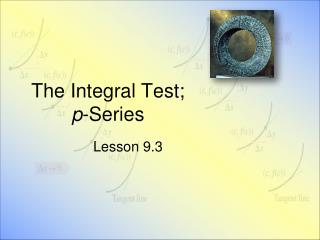Download PresentationThe Integral Test; p -Series

# The Integral Test; p -Series - PowerPoint PPT Presentation

The Integral Test; p -Series. Lesson 9.3. Divergence Test. Be careful not to confuse Sequence of general terms { a k } Sequence of partial sums { S k } We need the distinction for the divergence test If Then must diverge.I am the owner, or an agent authorized to act on behalf of the owner, of the copyrighted work described.
Download Presentation## The Integral Test; p -Series

Download Policy: Content on the Website is provided to you AS IS for your information and personal use and may not be sold / licensed / shared on other websites without getting consent from its author.While downloading, if for some reason you are not able to download a presentation, the publisher may have deleted the file from their server.

- - - - - - - - - - - - - - - - - - - - - - - - - - E N D - - - - - - - - - - - - - - - - - - - - - - - - - -
Presentation Transcript
1. The Integral Test; p-Series Lesson 9.3

2. Divergence Test • Be careful not to confuse • Sequence of general terms { ak } • Sequence of partial sums { Sk } • We need the distinction for the divergence test • If • Then must diverge Note this only tells us about divergence. It says nothing about convergence

3. Convergence Criterion • Given a series • If { Sk } is bounded above • Then the series converges • Otherwise it diverges • Note • Often difficult to apply • Not easy to determine { Sk } is bounded above

4. The Integral Test • Given ak = f(k) • k = 1, 2, … • f is positive, continuous, decreasing for x ≥ 1 • Then either • both converge … or • both diverge

5. Try It Out • Given • Does it converge or diverge? • Consider

6. p-Series • Definition • A series of the form • Wherep is a positive constant p-Series test • Converges if p > 1 • Diverges if 0 ≤ p ≤ 1

7. Try It Out • Given series • Use the p-series test to determine if it converges or diverges

8. Assignment • Lesson 9.3 • Page 620 • Exercises 1 - 35odd